# Release 12 Sidelink PSCCH and PSSCH Throughput

This example shows how to perform a Block Error Ratio (BLER) simulation of the 3GPP Release 12 sidelink control and shared channels in frequency-selective fading and Additive White Gaussian Noise (AWGN) using LTE Toolbox™.

### Introduction

3GPP Release 12 introduced the sidelink device-to-device communications for public safety in LTE. This example generates multiple Release 12 Physical Sidelink Control Channel (PSCCH) periods containing coded PSCCH and Physical Sidelink Shared Channel (PSSCH) transmissions. The resulting PSCCH period waveforms are then passed through a frequency-selective fading channel with AWGN, and the control channel and shared channel Block Error Ratios (BLERs) are calculated for a range of SNRs. The average number of transmission instances of the control and shared channels required for successful decoding at each SNR are also calculated. For information on how to model Release 14 V2X sidelink, check the following example: Release 14 V2X Sidelink PSCCH and PSSCH Throughput.

### Number of PSCCH Periods to Simulate

The example is executed for a simulation length of 5 PSCCH periods to keep the simulation time low. A large number of periods, `nPeriods`, should be used to produce statistically meaningful results.

```nPeriods = 5; ```

A frequency-selective fading channel is configured according to TS 36.101 Table 12.2.1-1 [ 1 ]. An EVA delay profile with a Doppler frequency of 70Hz and 2 receiver antennas with low correlation is used. The channel seed is specified so that each execution of this example will use the same fading process realization. The Rayleigh fading model uses random phase initialization and the output is normalized so that the average power is unity.

```channel.DelayProfile = 'EVA'; channel.DopplerFreq = 70.0; channel.NRxAnts = 2; channel.MIMOCorrelation = 'Low'; channel.Seed = 1; channel.ModelType = 'GMEDS'; channel.NTerms = 16; channel.NormalizeTxAnts = 'On'; channel.NormalizePathGains = 'On'; channel.InitPhase = 'Random'; ```

### SNR Configuration

Configure a range of SNR points, intended to cover both high and low BLER operating conditions.

```SNRIn = [-10.0 -5.0 0.0 5.0]; ```

### Channel Estimator Configuration

The variable `perfectChanEstimator` controls channel estimator behavior. Valid values are `true` or `false`. When set to `true` a perfect channel response is used as the estimate, otherwise an imperfect estimation based on the values of received Demodulation Reference Signals (DRS) is obtained. If `perfectChanEstimator` is set to `false`, a configuration structure `cec` is needed to parameterize the channel estimator.

```% Perfect channel estimator flag perfectChanEstimator = false; ```

The practical channel estimation configuration for the PSCCH and PSSCH channel estimators is defined below. A pilot averaging time window of 15 resource elements is used for both PSCCH and PSSCH, to ensure that the noise on the Demodulation Reference Signals (DRS) occurring in both slots is averaged. This averaging prioritizes pilot SNR improvement over accurate estimation of the channel time variation, which is reasonable as even at 70Hz Doppler frequency, the channel variation across a subframe is limited. A pilot averaging frequency window of 23 resource elements ensures that the noise on every DRS resource element across frequency is averaged for the PSCCH. This averaging prioritizes pilot SNR improvement over accurate estimation of the channel frequency selectivity, which is reasonable for the PSCCH as it is always QPSK modulated (so accuracy of channel estimation is not so critical) and it only occupies one resource block (the channel has limited frequency selectivity over this frequency span). For the PSSCH, this pilot averaging frequency window averages over roughly 2 resource blocks (10 resource blocks are allocated for the PSSCH for the parameters used in this example). Note that depending on the channel delay profile and Doppler frequency, the operating SNR, and the PSSCH modulation order and number of allocated resource blocks, different channel estimation parameters may give better performance for the PSSCH.

```cec.PilotAverage = 'UserDefined'; cec.TimeWindow = 15; cec.FreqWindow = 23; cec.InterpType = 'linear'; ```

### Configure BLER measurements

The logical variables `measureBLERForSCI` and `measureBLERForSLSCH` allow the BLER measurements and all related receiver processing to be disabled for the SCI and SL-SCH respectively. This allows the simulation to be configured to measure BLER for only one of the channels. Disabling the receiver processing for the other channel improves the execution speed.

```measureBLERForSCI = true; measureBLERForSLSCH = true; ```

### Create PSCCH Period Configuration

The class `PSCCHPeriod` represents the physical structure of a PSCCH period. See LTE Sidelink Resource Pools and PSCCH Period and PSCCHPeriod for more information.

```period = PSCCHPeriod; ```

### Resource Pool Configuration

A resource pool configuration for the transmission of D2D sidelink communication consists of a large number of parameters which the UE receives via Radio Resource Control (RRC) messages [ 3 ]. Alternatively, in the case of a UE operating out of network coverage, these parameters are pre-configured within the UE. In this simulation, reference resource pool configuration #1-FDD from TS 36.101 Annex A.7.2.1-1 [ 1 ] is used. These parameters can be set using the PSCCHPeriod.defaultConfig function by specifying the reference resource pool configuration number and bandwidth.

```% Configure the PSCCH period parameters for reference pool #1-FDD, 5MHz period.Config = PSCCHPeriod.defaultConfig(1,'5MHz'); % Set the control and data channel cyclic prefix length period.Config.sc_CP_Len_r12 = 'Normal'; period.Config.data_CP_Len_r12 = 'Normal'; ```

### Display Simulation Information

The variable `displaySimulationInformation` controls the display of simulation information such as the PSCCH resources used for each period, and whether or not SCI and SL-SCH decoding were successful.

```displaySimulationInformation = true; ```

### Display PSCCH Period

In order to illustrate the control and shared resource pools, the function period.displayPeriod is called which creates a plot showing the locations of the synchronization transmission (light blue), control resource pool (dark blue) and shared resource pool (yellow) within the PSCCH period.

```if (displaySimulationInformation) figure; period.displayPeriod; drawnow; end ```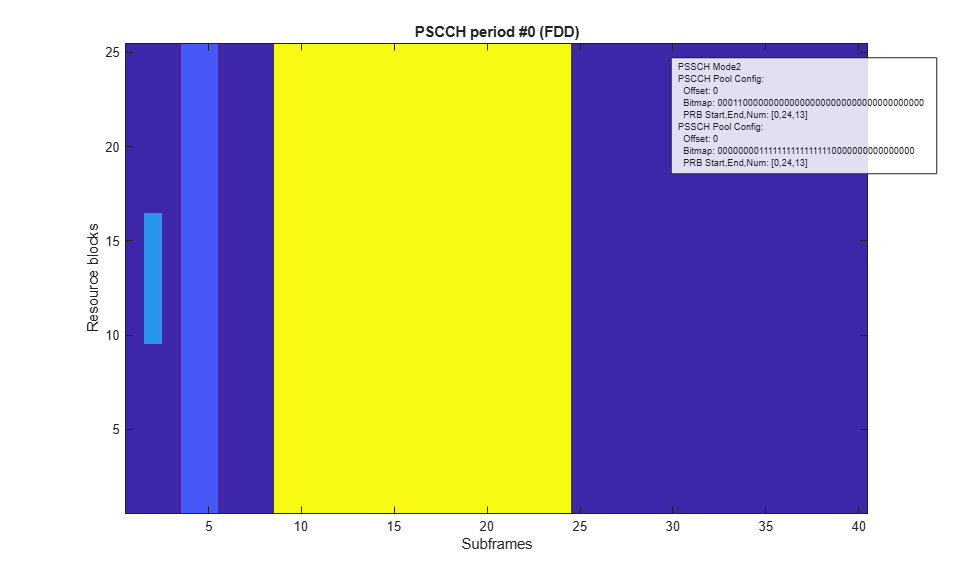### Create Sidelink Control Information (SCI) Message

A Sidelink Control Information (SCI) message `sciMessage` is created according to sidelink reference measurement channel CC.3 FDD defined in TS 36.101 [ 1 ] Annex A.6.4 and CD.1 FDD defined in Annex A.6.5 as follows:

• The Transport Block Size for CD.1 FDD (872), used for the shared channel transmission in this example, is assigned to the variable `TBS`.

• The number of allocated resource blocks for CD.1 FDD (10) is assigned to the variable `NPRB`, which will be used to ensure that the resource allocation for the shared channel contains the correct number of resource blocks. Note that the code rate for the SL-SCH is a function of the PSSCH bit capacity (arising from `NPRB` and the modulation order) and the transport block size `TBS`.

• The number of resource blocks `NSLRB` in the UE-specific settings structure `ue` is set equal to that configured in the PSCCH period, `period.Config.NSLRB`.

• Frequency hopping is enabled by setting `sciMessage.FreqHopping` to 1.

• The SCI message `sciMessage` is then created using the `lteSCI` function. Enabling the frequency hopping in the SCI message structure prior to calling `lteSCI` ensures that the `HoppingBits` field of `sciMessage.Allocation` is created.

• The `HoppingBits` field is set to 1, which in conjunction with the `NSLRB` value configures Type 2 hopping as described in TS 36.213 Section 8.4 [ 2 ].

• The `TimeResourcePattern` is set as defined in TS 36.101 Table A.6.4-1 [ 1 ].

• The `ModCoding` field is set to the value corresponding to the Transport Block Size `TBS`.

• The `NSAID` field is set to an arbitrary 8-bit value (a value in the range from 0 to 255), representing the least significant 8 bits of the ProSe Layer-2 Group ID. TS 36.101 Table A.6.5-1 [ 1 ] states that the Group destination ID is "as set by higher layers". The value used is recorded in the variable `expectedNSAID` and is the only part of the SCI message content that the UE is aware of prior to reception (the UE obtains it from higher layer signaling). In this example, the NSAID value stored in `expectedNSAID` will be used by the receiver, and the receiver will not access `sciMessage` at all.

```% Number of allocated resource blocks (NPRB) and Transport Block Size (TBS) % for RMC CD.1 FDD, TS 36.101 Table A.6.5-1 NPRB = 10; TBS = 872; % Create UE configuration and SCI message with frequency hopping enabled ue.NSLRB = period.Config.NSLRB; sciMessage.FreqHopping = 1; sciMessage.SCIFormat = 'Format0'; sciMessage = lteSCI(ue,sciMessage); % Set the hopping bits for Type 2 hopping and the time resource pattern for % RMC CC.3 FDD, TS 36.101 Table A.6.4-1 sciMessage.Allocation.HoppingBits = 1; sciMessage.TimeResourcePattern = 8; % Set the PSSCH MCS according to the TBS ITBSs = lteMCS(0:28,'PUSCH'); sciMessage.ModCoding = find(lteTBS(NPRB,ITBSs)==TBS,1,'first') - 1; % Set NSAID value, would be assigned by higher layers sciMessage.NSAID = 117; % Store NSAID value in 'expectedNSAID' so that the receiver won't have to % access 'sciMessage' at all expectedNSAID = sciMessage.NSAID; ```

### Create Set of Valid Resource Indicator Values

The set of Resource Indicator Values `RIVset` with the correct allocation size of 10 PRBs (given by RMC CD.1 FDD) are created. The period.getAllowedRIV function returns a vector of RIV values (`RIVset`) and a corresponding matrix of resource allocations (`range`) where each row contains the allocation length and starting resource block for each RIV. The `RIVset` vector is reduced to just the entries which have an allocation length equal to `NPRB` by performing logical indexing.

```[RIVset,range] = period.getAllowedRIV(sciMessage); RIVset = RIVset(range(:,1) == NPRB); ```

### Display the PSCCH Period Including PSSCH Resources Selected by SCI Message

An example of the actual PSCCH and PSSCH subframes and resource blocks for transmission will be plotted. The SCI message is configured with the first PSCCH resource value `PSCCHResource=0` and the first PSSCH RIV value `Allocation.RIV = RIVset(1)`. The function period.displayPeriod is called, passing in the SCI message, and the sets of PSCCH and PSSCH subframes and resource blocks that will actually be used for transmission are plotted. The subframes and resource blocks are colored green, and are a subset of the overall control and shared resource pool, shown in blue and yellow respectively. See the LTE Sidelink Resource Pools and PSCCH Period example for more information. In accordance with the definition in TS 36.101 Table 12.2.1-1 [ 1 ], the RIV value transmitted in the SCI message (which determines the PSSCH transmission resources within the pool) will be selected randomly (uniformly) from the set `RIVset`, for each PSCCH period. Similarly, the PSCCH resource value (which determines the PSCCH transmission resources within the pool) will be selected randomly (uniformly) across its valid range from 0 to `NumPSCCHReource-1`, for each PSCCH period.

```% Select the first PSCCH and PSSCH resource sciMessage.PSCCHResource = 0; sciMessage.Allocation.RIV = RIVset(1); % Display the subframes and resource blocks for transmission if (displaySimulationInformation) figure; period.displayPeriod(sciMessage); drawnow; end ```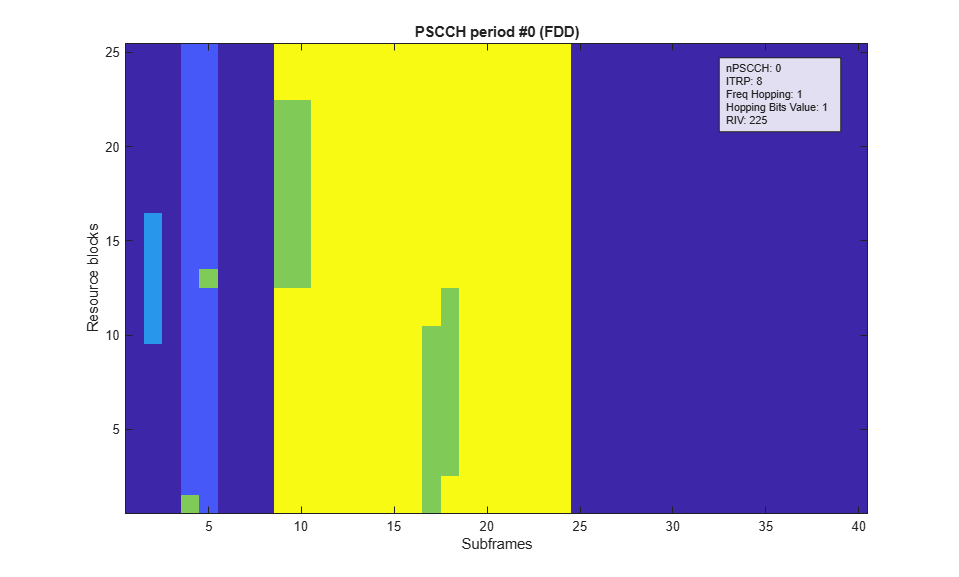### Select Receiver Behavior When SCI Decoding Fails

The logical variable `sciAssumed` controls the simulation behavior in terms of the effect the control channel decoding has on the shared channel decoding. If `sciAssumed` is true, the receiver will assume that the SCI message was correctly decoded when attempting shared channel reception. This allows the performance of the shared channel reception to be measured independently from the performance of the control channel reception. If `sciAssumed` is false, a control channel decoding failure implies a shared channel decoding failure. Note that if the BLER measurement for the SCI is disabled above (`measureBLERForSCI=false`), and the BLER measurement for the SL-SCH is enabled (`measureBLERForSLSCH=true`), the receiver will assume that the SCI message was correctly decoded when measuring SL-SCH BLER regardless of the setting of `sciAssumed` here.

```sciAssumed = true; ```

### Create Variables to Record Simulated Performance

The performance of the receiver is recorded in the matrices `controlErrors` and `sharedErrors`. Each matrix has a row for each SNR point and a column for each PSCCH period simulated. The elements will be set to 1 for SNR points/PSCCH periods where the respective channel combination (PSCCH and SCI or PSSCH and SL-SCH) fails to decode successfully. Additionally, vectors `controlRxs` and `sharedRxs` record the number of transmission instances that were combined before successful decoding in each period. Note that the number of transmission instances is 2 for the control channel and 4 for the shared channel. The vectors `controlRxs` and `sharedRxs` are initialized with these maximum values, which will be the values returned in periods where the control and shared channel decoding fail.

```controlErrors = zeros(length(SNRIn),nPeriods); sharedErrors = zeros(length(SNRIn),nPeriods); controlRxs = ones(length(SNRIn),nPeriods) * 2; sharedRxs = ones(length(SNRIn),nPeriods) * 4; ```

### Processing

For each PSCCH period, the following operations are performed:

• Update the PSCCH period number: The field `NPSCCHPeriod` of the period configuration is updated to match the currently transmitted period. This field is used in conjunction with the Sidelink Control (SC) period `sc_Period_r12` and offset indicator `sc_TF_ResourceConfig_r12.offsetIndicator_r12` to determine the Direct Frame Number (DFN) and subframe number for each subframe in the waveform.

• Choose a random PSCCH resource: For a sidelink UE in transmission mode 2 (e.g. out-of-coverage), the PSCCH resource `PSCCHResource` must be chosen randomly according to a uniform distribution between 0 and `period.NumPSCCHReource-1`. The PSCCH resource will select a random pair of subframes and Physical Resource Blocks (PRBs) in the PSCCH resource pool in which to transmit the two transmission instances of the SCI message. The `PSCCHResource` is added to the SCI message structure in order to pass it into the period.generateWaveform function. Note that for an in-coverage UE (i.e. operating in transmission mode 1), the control information is given by a DCI format 5 message sent by the eNodeB, which includes a `PSCCHResource` chosen by the eNodeB.

• Choose a random PSSCH resource: As given by the definition in TS 36.101 Table 12.2.1-1 [ 1 ], the Resource Indicator Value (RIV) transmitted in the SCI message will be selected randomly (uniformly) from the set `RIVset`, the RIVs whose number of allocated PRBs matches the configured RMC CD.1 FDD.

• Generate PSCCH period waveform: The PSCCH period waveform is generated by the period.generateWaveform function using its configuration parameters and the content of the SCI message passed in, plus the `PSCCHResource` field added to the message in the previous step.

• Apply frequency-selective fading: The PSCCH period waveform is passed through a frequency-selective fading channel using the EVA delay profile with a Doppler frequency of 70Hz and 2 receiver antennas with low correlation, as described in TS 36.101 Table 12.2.1-1 [ 1 ].

• Add AWGN: AWGN is added to achieve the specified SNR per resource element.

• Receive the control and shared channels: The receiver processing consists of PSCCH demodulation, SCI decoding, PSSCH demodulation and SL-SCH decoding. These steps are described in more detail below.

Note that the "Add AWGN" and "Receive control and shared channels" steps are repeated for each SNR point using the same faded waveform.

The receiver performs the following steps:

• Synchronize and SC-FDMA demodulate each subframe in the PSCCH subframe pool: Before attempting PSCCH demodulation and SCI decoding for each PSCCH resource, every subframe in the PSCCH subframe pool is SC-FDMA demodulated. For each subframe, the appropriate subframe of the waveform is synchronized using `lteSLFrameOffsetPSCCH`. The PSCCH resource used in the transmitter is unknown, therefore the PRB used by the PSCCH in the subframe is unknown, so the synchronization is performed for each PRB in the PSCCH resource block pool and the timing offset for the correlation with the strongest peak is used. The synchronized waveform is then SC-FDMA demodulated using `lteSLSCFDMADemodulate`. Since the number of subframes in the PSCCH pool is likely to be small compared to the total number of PSCCH resource values (with multiple PSCCH resource values corresponding to the same subframe in the pool), it is more efficient to cache the SC-FDMA demodulated subframes and use the resulting resource grids indicated by the PSCCH subframe resources for a given PSCCH resource value. Therefore, the waveform is SC-FDMA demodulated outside of the PSCCH resource value loop. Note that the approach of choosing the timing offset corresponding to the PRB with the strongest correlation peak assumes that only one PSCCH is being transmitted. A more robust (but more expensive) approach would be to perform timing synchronization and SC-FDMA demodulation for each PSCCH resource.

For each PSCCH resource value until the SCI is decoded:

• Get the PSCCH resources: Within a period, the PSCCH is transmitted twice for a configured PSCCH resource `pscchResource`. The function period.getPSCCHResources provides the two subframes that carry PSCCH and corresponding PRBs allocated to PSCCH within those subframes. The subframe numbers are used to extract the appropriate subframes from the PSCCH period waveform, and the PRBs are used to configure the PSCCH resource extraction within those subframes. Note that the PSCCH will not be transmitted in synchronization subframes.

For each PSCCH transmission instance for the current PSCCH resource value:

• Perform PSCCH channel estimation: PSCCH channel estimation is performed using `lteSLChannelEstimatePSCCH` or the local function `perfectChannelEstimate` depending on the value of `perfectChanEstimator`. The channel estimator also produces an estimate of the noise power which can be used for MMSE equalization.

• Perform PSCCH equalization: The PSCCH symbols are MMSE equalized using `lteEqualizeMMSE` with the channel estimate and noise estimate obtained above.

• Perform PSCCH demodulation: The equalized PSCCH symbols are demodulated using `ltePSCCHDecode`. This function performs the inversion of the transmitter modulation steps (SC-FDMA transform deprecoding, QPSK symbol demodulation and descrambling).

• Perform SCI decoding: SCI decoding is attempted using `lteSCIDecode`. The number of original information bits in the SCI message is given by `lteSCIInfo`. If the decoded CRC is zero, the decoded message bits are converted into the corresponding message structure using `lteSCI`. The `NSAID` message field, which is the eight LSBs of the group destination ID, is compared with the expected NSAID value. If they are equal, the SCI decoding is considered successful and the PSCCH resource value loop is terminated. The number of required transmission instances (1 or 2) is recorded in `controlRxs`.

The behavior of the shared channel decoding with respect to a failed SCI decoding is controlled by the variable `sciAssumed`. If `sciAssumed` is false, the failed SCI decoding immediately implies a failure to decode the SL-SCH and no further processing takes place for the current PSCCH period. If `sciAssumed` is true, the transmitted SCI message is assumed to be known to the receiver and will be used in place of the received SCI message. If `sciAssumed` is true or if SCI decoding was successful, the receiver proceeds with SL-SCH decoding. Note that if SCI BLER measurement is disabled but SL-SCH BLER measurement is enabled then the transmitted SCI message is assumed to be known to the receiver.

The PSSCH / SL-SCH is transmitted four times, using HARQ where four redundancy versions (RV) with the sequence [0 2 3 1] are transmitted. There is no HARQ feedback as the sidelink communication transmissions are for a group of UEs (not for an individual receiving UE).

For each PSSCH transmission instance until the SL-SCH is decoded:

• Get the PSSCH resources: The function period.getPSSCHResources provides a vector of the subframes within the period that carry PSSCH and a matrix containing the PRB sets allocated to PSSCH within those subframes for the PSSCH configuration given by the decoded SCI message. This is similar to the resources described above for the PSCCH, with the difference being that the PSSCH can be sent on multiple PRBs but the PSCCH is only sent on a single PRB. As with the PSCCH, the subframe numbers are used to extract the appropriate subframes from the PSCCH period waveform, and the PRBs are used to configure the PSSCH resource extraction within those subframes. Additionally, period.getPSSCHResources returns a vector of PSCCH subframe numbers, which are the subframe numbers within the PSSCH resource pool for which this PSSCH is transmitted. These values are used to configure the descrambling of the PSSCH in each subframe. Since the PSCCH is not transmitted in synchronization subframes, any PSCCH subframes that correspond to synchronization subframes are configured to be skipped, by removing them from the vectors `sf` and `prb`. Prior to skipping any synchronization subframes, the redundancy version indices `IRV` for SL-SCH decoding are calculated for each resource. These indices must be computed prior to the skipping of any synchronization subframes because the index sequence is related solely to the position of the PSSCH subframes within the subframe pool.

• Synchronize and SC-FDMA demodulate subframe carrying PSSCH: The appropriate subframe of the waveform is synchronized using `lteSLFrameOffsetPSSCH`. The synchronized waveform is SC-FDMA demodulated using `lteSLSCFDMADemodulate`. Note that unlike the timing synchronization and SC-FDMA demodulation for the PSCCH, the synchronization and demodulation for the PSSCH is carried out for each transmission instance (whereas for the PSCCH, all subframes in the PSCCH resource pool were demodulated first). The reason is that unlike the PSCCH, the PSSCH transmission instances occur in distinct subframes and those subframes are likely to be a small subset of the overall PSSCH subframe pool. Therefore, it is efficient to only synchronize and SC-FDMA demodulate the subframes corresponding to a PSSCH transmission instance.

• Perform PSSCH channel estimation: PSSCH channel estimation is performed using `lteSLChannelEstimatePSSCH` or the local function `perfectChannelEstimate` depending on the value of `perfectChanEstimator`. The channel estimator also produces an estimate of the noise power which can be used for MMSE equalization.

• Perform PSSCH equalization: The PSSCH symbols are MMSE equalized using `lteEqualizeMMSE` with the channel estimate and noise estimate obtained above.

• Perform PSSCH demodulation: The equalized PSSCH symbols are demodulated using `ltePSSCHDecode`. This function performs the inversion of the transmitter modulation steps (SC-FDMA transform deprecoding, QPSK or 16QAM symbol demodulation and descrambling).

• Perform SL-SCH decoding: The received redundancy versions are combined and decoded using `lteSLSCHDecode`. If the SL-SCH CRC is zero, the HARQ combining loop is terminated and the SL-SCH decoding is successful. The number of required redundancy versions (from 1 to 4) is recorded in `sharedRxs`.

```% If SCI BLER is not being measured, 'sciAssumed' must be set so that the % receiver can assume knowledge of the SCI if (~measureBLERForSCI) sciAssumed = true; end rng('default'); % Repeat for each PSCCH period p = 1; while (p<=nPeriods) % Update PSCCH period number period.Config.NPSCCHPeriod = p - 1; % Choose a random PSCCH resource sciMessage.PSCCHResource = randi([0 period.NumPSCCHResource-1]); % Choose a random PSSCH resource sciMessage.Allocation.RIV = RIVset(randi(length(RIVset))); if (displaySimulationInformation) fprintf('\nSimulating PSCCH period %d of %d\n',p,nPeriods); fprintf('Randomly selected PSCCH resource = %d\n',sciMessage.PSCCHResource); fprintf('Randomly selected PSSCH RIV = %d\n',sciMessage.Allocation.RIV); end % Generate PSCCH period waveform txWaveform = period.generateWaveform(sciMessage); % Set the sampling rate and init time for frequency-selective fading ue.CyclicPrefixSL = period.Config.sc_CP_Len_r12; info = lteSLSCFDMAInfo(ue); channel.SamplingRate = info.SamplingRate; channel.InitTime = (p-1)*period.Config.sc_Period_r12*0.001; periodInitTime = channel.InitTime; % Cache the period init time % Apply frequency-selective fading [fadedWaveform, fadingInfo] = lteFadingChannel(channel,txWaveform); % For each SNR point, add AWGN and receive control and shared channels for i = 1:length(SNRIn) if (displaySimulationInformation && (measureBLERForSCI || measureBLERForSLSCH)) fprintf('Receiving at %gdB SNR\n',SNRIn(i)); end % Add AWGN SNR = 10^(SNRIn(i)/20); N = 1/(SNR*sqrt(double(info.Nfft)))/sqrt(2.0); noise = N*complex(randn(size(fadedWaveform)), randn(size(fadedWaveform))); rxWaveform = fadedWaveform + noise; % Create PSCCH receiver configuration pscch.SidelinkMode = 'D2D'; pscch.NSLRB = period.Config.NSLRB; pscch.CyclicPrefixSL = period.Config.sc_CP_Len_r12; % Establish the number of time-domain samples 'Nt' per subframe and % number of SC-FDMA symbols 'L' per subframe pscchGridInfo = lteSLSCFDMAInfo(pscch); Nt = pscchGridInfo.SamplingRate * 1e-3; L = numel(pscchGridInfo.CyclicPrefixLengths); % If SCI BLER measurement is configured: sciDecoded = false; if (measureBLERForSCI) % Synchronize and extract each subframe of the PSCCH subframe % pool from the received waveform and perform SC-FDMA % demodulation. The timing offset used is the offset % corresponding to the strongest correlation peak when % correlating with the PSCCH DRS for each PRB in the PSCCH % subframe pool nSubframes = period.Config.sc_Period_r12; pscchPoolGrid = repmat(lteSLResourceGrid(pscch),1,nSubframes,size(rxWaveform,2)); offsetpool = []; % Variable to store the offset for each PSCCH subframe for l = period.PSCCHSubframePool if(perfectChanEstimator) offset = hPerfectTimingEstimate(fadingInfo); else subframeWaveform = rxWaveform(l*Nt + (1:Nt),:); bestCorr = 0.0; for prb = period.PSCCHResourceBlockPool.' pscch.PRBSet = prb; [thisoffset,thiscorr] = lteSLFrameOffsetPSCCH(pscch,subframeWaveform); % Find the best correlation across all antennas and % store the corresponding offset maxCorr = max(thiscorr(:)); if maxCorr > bestCorr bestCorr = maxCorr; offset = max(0,thisoffset); % No negative offset end end end subframe = lteSLSCFDMADemodulate(pscch,rxWaveform(l*Nt + offset + (1:Nt),:)); pscchPoolGrid(:,l*L + (1:L),:) = subframe; offsetpool = [offsetpool offset]; %#ok<AGROW> end % Repeat for each PSCCH resource until the SCI is decoded pscchResource = 0; while (pscchResource < period.NumPSCCHResource && ~sciDecoded) % Get the PSCCH subframes 'sf' and PRB allocations 'prb' % for the current PSCCH resource [sf,prb] = period.getPSCCHResources(pscchResource); % Remove any resources that overlap with synchronization % subframes. PSCCH will not be transmitted in these % subframes [~,syncIndex] = intersect(sf,period.SyncSubframes); sf(syncIndex) = []; prb(syncIndex) = []; % Repeat for each PSCCH transmission instance until the SCI % is decoded tx = 1; while (tx <= min(length(sf),2) && ~sciDecoded) % Configure the PSCCH receiver for the PRB allocation pscch.PRBSet = prb(:,tx); % Select the appropriate subframe resource grid from % the PSCCH subframe pool subframe = pscchPoolGrid(:,sf(tx)*L + (1:L),:); % Perform channel estimation if(perfectChanEstimator) % Update the init time to correspond to the current % subframe channel.InitTime = periodInitTime + (sf(tx)*Nt/channel.SamplingRate); [hest,nest] = perfectChannelEstimate(pscch,channel,noise,offsetpool(tx)); else [hest,nest] = lteSLChannelEstimatePSCCH(pscch,cec,subframe); end % Extract the received PSCCH symbols and the % corresponding channel estimate, and perform % equalization [pscchIndices,pscchIndicesInfo] = ltePSCCHIndices(pscch); [pscchRx,pscchHest] = lteExtractResources(pscchIndices,subframe,hest); pscchSymbols = lteEqualizeMMSE(pscchRx,pscchHest,nest); % If we are receiving the first PSCCH transmission % instance, reset the receiver buffer if (tx==1) codedSciBits = zeros(pscchIndicesInfo.G,1); end % Demodulate the PSCCH and add the result into the % receiver buffer codedSciBits = codedSciBits + ltePSCCHDecode(pscchSymbols); % Decode the SCI message. If successful (CRC=0), check % the NSAID field of the decoded message against the % transmitted message sciInfo = lteSCIInfo(pscch); [sciBits,sciCRC] = lteSCIDecode(sciInfo.Format0,codedSciBits); if (sciCRC==0) sciMessageRx = lteSCI(pscch,sciBits); if (sciMessageRx.NSAID==expectedNSAID) sciDecoded = true; controlRxs(i,p) = tx; if (displaySimulationInformation) fprintf(' SCI decoded, transmissions combined = %d\n',tx); end else if (displaySimulationInformation) fprintf(' SCI decoded, but NSAID value (%d) did not match expected value (%d)\n',sciMessageRx.NSAID,expectedNSAID); end end end % Increment the PSCCH transmission instance index tx = tx + 1; end % Increment the PSCCH resource number pscchResource = pscchResource + 1; end end % If SCI decoding failed and the SCI is assumed for SL-SCH % decoding, set the decoded SCI message equal to the transmitted % SCI message if (~sciDecoded) if (displaySimulationInformation && measureBLERForSCI) fprintf(' SCI decoding failed\n'); end if (sciAssumed) sciMessageRx = sciMessage; end end % If SL-SCH BLER measurement is configured and if the SCI was % successfully decoded or if SCI decoding success is assumed, % perform PSSCH reception and SL-SCH decoding slschDecoded = false; if (measureBLERForSLSCH && (sciDecoded || sciAssumed)) % Create PSSCH receiver configuration pssch.SidelinkMode = 'D2D'; pssch.NSLRB = period.Config.NSLRB; pssch.CyclicPrefixSL = period.Config.data_CP_Len_r12; pssch.NTurboDecIts = 5; % Establish the number of time-domain samples 'Nt' per subframe psschGridInfo = lteSLSCFDMAInfo(pssch); Nt = psschGridInfo.SamplingRate * 1e-3; % Get the PSSCH subframes 'sf', PRB allocations 'prb' and PSSCH % subframe numbers 'nsf' for the configuration given by the % decoded SCI message. 'sf' are the subframe numbers within the % SC period, 'nsf' are the subframe numbers within the PSSCH % subframe pool [sf,prb,nsf] = period.getPSSCHResources(sciMessageRx); % Create the redundancy version index sequence (IRV) % corresponding to the PSSCH transmission instances. This must % be computed "up front" prior to the removal of any % synchronization subframes in the next step, otherwise the RV % sequence is lost IRV = 0:length(sf)-1; % Remove any resources that overlap with synchronization % subframes. PSSCH will not be transmitted in these subframes [~,syncIndex] = intersect(sf,period.SyncSubframes); sf(syncIndex) = []; prb(:,syncIndex) = []; nsf(syncIndex) = []; IRV(syncIndex) = []; % Configure the PSSCH receiver NSAID value and modulation from % the decoded SCI message, and establish the SL-SCH transport % block size (TBS) pssch.NSAID = sciMessageRx.NSAID; [ITBS,modulation] = lteMCS(sciMessageRx.ModCoding,'PUSCH'); if (strcmpi(modulation,'64QAM')) modulation = '16QAM'; end pssch.Modulation = modulation; NPRB = max(size(prb,1),1); TBS = lteTBS(NPRB,ITBS); % Repeat for each PSSCH transmission instance until the SL-SCH % is decoded tx = 1; slschDecState = []; rvsequence = [0 2 3 1]; while (tx <= min(length(sf),4) && ~slschDecoded) % Configure the PSSCH receiver for the PSSCH subframe % number, PRB allocation and redundancy version (RV). If % the RV index is zero (corresponding to the first PSSCH % transmission instance in a block of 4 transmissions), % reset the receiver buffer pssch.NSubframePSSCH = nsf(tx); pssch.PRBSet = prb(:,tx); pssch.RV = rvsequence(mod(IRV(tx),4)+1); if (IRV(tx)==0) slschDecState = []; end % Perform timing synchronization, extract the appropriate % subframe of the received waveform, and perform SC-FDMA % demodulation if(perfectChanEstimator) offset = hPerfectTimingEstimate(fadingInfo); else offset = lteSLFrameOffsetPSSCH(pssch,rxWaveform(sf(tx)*Nt + (1:Nt),:)); offset = max(0,offset); % No negative offset end subframeWaveform = rxWaveform(sf(tx)*Nt + offset + (1:Nt),:); subframe = lteSLSCFDMADemodulate(pssch,subframeWaveform); % Perform channel estimation, extract the received PSSCH % symbols and the corresponding channel estimate, and % perform equalization if(perfectChanEstimator) % Update the init time to correspond to the current % subframe channel.InitTime = periodInitTime + (sf(tx)*Nt/channel.SamplingRate); [hest,nest] = perfectChannelEstimate(pssch,channel,noise,offset); else [hest,nest] = lteSLChannelEstimatePSSCH(pssch,cec,subframe); end psschIndices = ltePSSCHIndices(pssch); [psschRx,psschHest] = lteExtractResources(psschIndices,subframe,hest); psschSymbols = lteEqualizeMMSE(psschRx,psschHest,nest); % Demodulate the PSSCH codedSlschBits = ltePSSCHDecode(pssch,psschSymbols); % Decode the SL-SCH including soft combining into the % receiver buffer and check the CRC [~,slschCRC,slschDecState] = lteSLSCHDecode(pssch,TBS,codedSlschBits,slschDecState); if (slschCRC==0) slschDecoded = true; sharedRxs(i,p) = tx; if (displaySimulationInformation) fprintf(' SL-SCH decoded, transmissions combined = %d\n',tx); end end % Increment the PSSCH transmission instance index tx = tx + 1; end end % Record control and shared channel errors controlErrors(i,p) = ~sciDecoded; sharedErrors(i,p) = ~slschDecoded; if (displaySimulationInformation && ~slschDecoded && measureBLERForSLSCH) fprintf(' SL-SCH decoding failed\n'); end end % Update the PSCCH period number p = p + 1; end ```
```Simulating PSCCH period 1 of 5 Randomly selected PSCCH resource = 19 Randomly selected PSSCH RIV = 239 Receiving at -10dB SNR SCI decoding failed SL-SCH decoding failed Receiving at -5dB SNR SCI decoded, transmissions combined = 2 SL-SCH decoded, transmissions combined = 2 Receiving at 0dB SNR SCI decoded, transmissions combined = 1 SL-SCH decoded, transmissions combined = 1 Receiving at 5dB SNR SCI decoded, transmissions combined = 1 SL-SCH decoded, transmissions combined = 1 Simulating PSCCH period 2 of 5 Randomly selected PSCCH resource = 12 Randomly selected PSSCH RIV = 238 Receiving at -10dB SNR SCI decoding failed SL-SCH decoded, transmissions combined = 4 Receiving at -5dB SNR SCI decoding failed SL-SCH decoded, transmissions combined = 2 Receiving at 0dB SNR SCI decoded, transmissions combined = 1 SL-SCH decoded, transmissions combined = 1 Receiving at 5dB SNR SCI decoded, transmissions combined = 1 SL-SCH decoded, transmissions combined = 1 Simulating PSCCH period 3 of 5 Randomly selected PSCCH resource = 10 Randomly selected PSSCH RIV = 226 Receiving at -10dB SNR SCI decoding failed SL-SCH decoded, transmissions combined = 4 Receiving at -5dB SNR SCI decoded, transmissions combined = 2 SL-SCH decoded, transmissions combined = 1 Receiving at 0dB SNR SCI decoded, transmissions combined = 1 SL-SCH decoded, transmissions combined = 1 Receiving at 5dB SNR SCI decoded, transmissions combined = 1 SL-SCH decoded, transmissions combined = 1 Simulating PSCCH period 4 of 5 Randomly selected PSCCH resource = 1 Randomly selected PSSCH RIV = 237 Receiving at -10dB SNR SCI decoded, transmissions combined = 1 SL-SCH decoding failed Receiving at -5dB SNR SCI decoded, transmissions combined = 1 SL-SCH decoded, transmissions combined = 2 Receiving at 0dB SNR SCI decoded, transmissions combined = 1 SL-SCH decoded, transmissions combined = 1 Receiving at 5dB SNR SCI decoded, transmissions combined = 1 SL-SCH decoded, transmissions combined = 1 Simulating PSCCH period 5 of 5 Randomly selected PSCCH resource = 15 Randomly selected PSSCH RIV = 237 Receiving at -10dB SNR SCI decoding failed SL-SCH decoding failed Receiving at -5dB SNR SCI decoding failed SL-SCH decoded, transmissions combined = 3 Receiving at 0dB SNR SCI decoded, transmissions combined = 1 SL-SCH decoded, transmissions combined = 2 Receiving at 5dB SNR SCI decoded, transmissions combined = 1 SL-SCH decoded, transmissions combined = 1 ```

### Calculate Control Channel and Shared Channel BLER

The overall BLER for the control channel (PSCCH and SCI) and shared channel (PSSCH and SL-SCH) are computed by averaging the `controlErrors` and `sharedErrors` matrices along their second dimension i.e. averaging each row, to produce vectors `controlBLER` and `sharedBLER` containing the BLER values for each SNR point tested. Similarly, `controlRxs` and `sharedRxs` are averaged to produce vectors `controlRxsAvg` and `sharedRxsAvg` containing the average number of transmission instances that needed to be combined for successful reception.

```if (measureBLERForSCI) controlBLER = 100*mean(controlErrors,2); controlRxsAvg = mean(controlRxs,2); else controlBLER = []; controlRxsAvg = []; end if (measureBLERForSLSCH) sharedBLER = 100*mean(sharedErrors,2); sharedRxsAvg = mean(sharedRxs,2); else sharedBLER = []; sharedRxsAvg = []; end ```

### Plot Results

Finally the test results are plotted. The BLER for the control channel and shared channel are plotted for each SNR point. The average number of transmission instances that need to be combined for successful reception for each SNR are also plotted. Note that the number of transmission instances is 2 for the control channel and 4 for the shared channel.

```if (measureBLERForSCI || measureBLERForSLSCH) plotResults(channel,SNRIn,controlBLER,sharedBLER,controlRxsAvg,sharedRxsAvg); end ```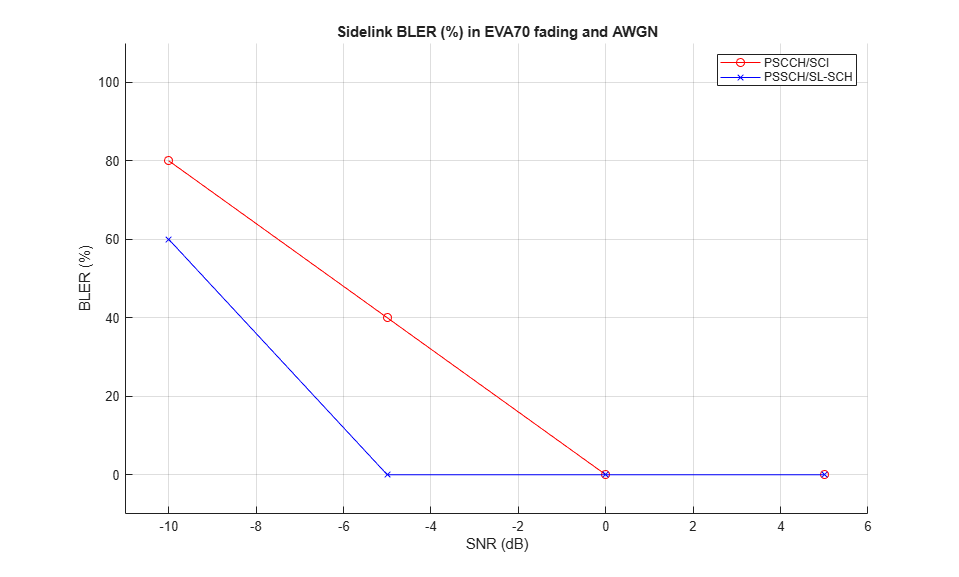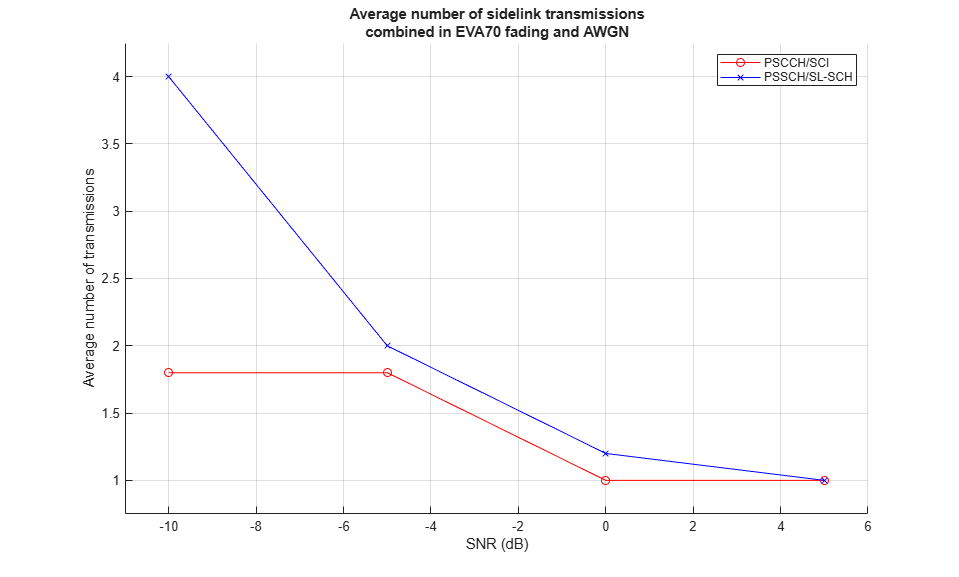Since the generated plots were obtained with a low number of PSCCH periods the results shown are not representative. A 1000 PSCCH period simulation with practical channel estimation including additional SNR points produced the results shown below.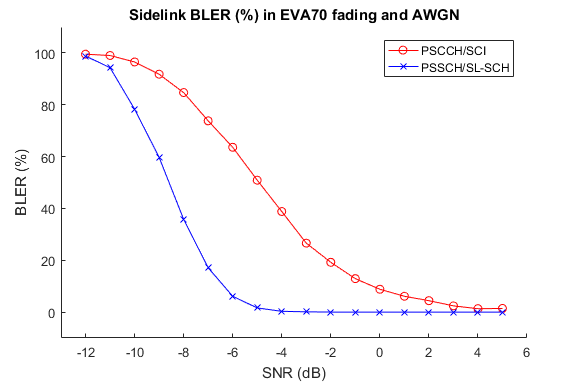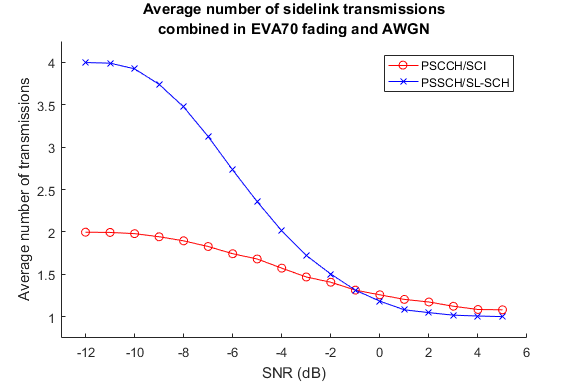### Appendix

This example uses the helper function:

### Selected Bibliography

1. 3GPP TS 36.101 "User Equipment (UE) radio transmission and reception"

2. 3GPP TS 36.213 "Physical layer procedures"

3. 3GPP TS 36.331 "Radio Resource Control (RRC) Protocol specification"

### Local Functions

The following local functions are used in this example:

• `perfectChannelEstimate`: perfect channel estimation

• `plotResults`: plot the results of the example

```% Calculate the perfect channel estimate and the noise estimate function [hest,nest] = perfectChannelEstimate(rxConfig,channel,noise,frameOffset) % Use the uplink perfect channel estimator to calculate % the sidelink estimate as both use SC-FDMA rxConfig.NTxAnts = 1; rxConfig.NULRB = rxConfig.NSLRB; rxConfig.CyclicPrefixUL = rxConfig.CyclicPrefixSL; rxConfig.TotSubframes = 1; hest = lteULPerfectChannelEstimate(rxConfig,channel,frameOffset); noiseGrid = lteSLSCFDMADemodulate(rxConfig,noise(1+frameOffset:end,:)); nest = var(noiseGrid(:)); end function plotResults(channel,SNRIn,controlBLER,sharedBLER,controlRxsAvg,sharedRxsAvg) fadingDescription = sprintf('%s%s',channel.DelayProfile,num2str(channel.DopplerFreq)); figure; hold on; legends = {}; if (~isempty(controlBLER)) plot(SNRIn,controlBLER,'ro-'); legends = [legends 'PSCCH/SCI']; end if (~isempty(sharedBLER)) plot(SNRIn,sharedBLER,'bx-'); legends = [legends 'PSSCH/SL-SCH']; end legend(legends); title(sprintf('Sidelink BLER (%%) in %s fading and AWGN',fadingDescription)); ylabel('BLER (%)'); xlabel('SNR (dB)'); axis([SNRIn(1)-1 SNRIn(end)+1 -10 110]); grid on figure; hold on; if (~isempty(controlBLER)) plot(SNRIn,controlRxsAvg,'ro-'); end if (~isempty(sharedBLER)) plot(SNRIn,sharedRxsAvg,'bx-'); end legend(legends); title(sprintf('Average number of sidelink transmissions\ncombined in %s fading and AWGN',fadingDescription)); ylabel('Average number of transmissions'); xlabel('SNR (dB)'); axis([SNRIn(1)-1 SNRIn(end)+1 0.75 4.25]) grid on end ```